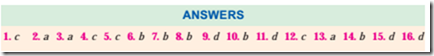# Objective test on transformer and three phase.

OBJECTIVE TEST

1. Which of the following connections is best suited for 3-phase, 4-wire service ?
(a) ∆ − ∆ (b) Y − Y
(c) ∆ − Y (d) Y − ∆
2. In a three-phase Y-Y transformer connection, neutral is fundamental to the
(a) suppression of harmonics
(b) passage of unbalanced currents due to
(c) provision of dual electric service
(d) balancing of phase voltages with respect to line voltages.
3. As compared to ∆ − ∆ bank, the capacity of the V − V bank of transformers is ……… percent.
(a) 57.7 (b) 66.7
(c) 50 (d) 86.6
4. If three transformers in a ∆ − ∆ are delivering their rated load and one transformer is removed, then overload on each of the remaining transformers is ……… percent.
(a) 66.7 (b) 173.2
(c) 73.2 (d) 58
5. When a V − V system is converted into a ∆ − ∆ system, increase in capacity of the system is ……… percent.
(a) 86.6 (b) 66.7
(c) 73.2 (d) 50
6. For supplying a balanced 3 − φ load of 40-kVA, rating of each transformer in V − V bank should be nearly ……… kVA.
(a) 20 (b) 23
(c) 34.6 (d) 25
7. When a closed − ∆ bank is converted into an open − ∆ bank, each of the two remaining transformers supplies ……… percent of the original load.
(a) 66.7 (b) 57.7
(c) 50 (d) 73.2
8. If the load p.f. is 0.866, then the average p.f. of the V – V bank is
(a) 0.886 (b) 0.75
(c) 0.51 (d) 0.65
9. A T − T connection has higher ratio of utilization that a V − V connection only when

(a) identical transformers are used
(c) load power factor is unity
(d) non-identical transformers are used.
10. The biggest advantage of T – T connection over the V – V connection for 3-phase power transformation is that it provides
(a) a set of balanced voltages under load
(b) a true 3-phase, 4-wire system
(c) a higher ratio of utilization
(d) more voltages.
11. Of the following statements concerning parallel operation of transformers, the one which is not correct is
(a) transformers must have equal voltage
ratings
(b) transformers must have same ratio of transformation
(c) transformers must be operated at the same
frequency
(d) transformers must have equal kVA ratings.

<br class="Apple-in

12. Statement
An auto-transformer is more efficient in transferring energy from primary to secondary circuit.
Reason
Because it does so both inductively and
conductively.
Key
(a) statement is false, reason is correct and
relevant
(b) statement is correct, reason is correct but
irrelevant
(c) both statement and reason are correct and
are connected to each other as cause and
effect
(d) both statement and reason are false.
13. Out of the following given choices for poly phase transformer connections which one will you select for three-to-two phase conversion ?
(a) Scott (b) star/star
(c) double Scott (d) star/double-delta
14. A T − T transformer cannot be paralleled with …….. transformer.
(a) V − V (b) Y − ∆
(c) Y − Y (d) ∆ − ∆
15. Instrument transformers are used on a.c. circuits for extending the range of
(a) ammeters (b) voltmeters
(c) wattmeters (d) all of the above.
16. Before removing the ammeter from a current transformer, its secondary must be shortcircuited in order to avoid
(a) excessive heating of the core
(b) high secondary e.m.f.
(c) increase in iron losses
(d) all of the above#### Incoming search terms: